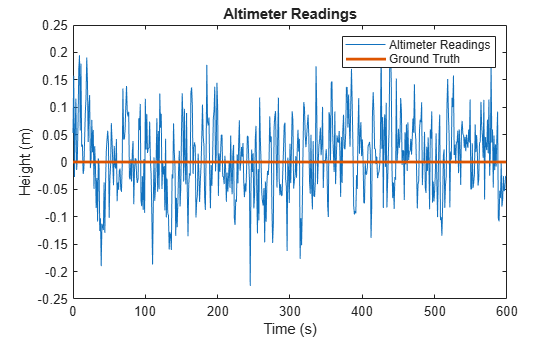Main Content

# altimeterSensor

Altimeter simulation model

## Description

The `altimeterSensor` System object™ models receiving data from an altimeter sensor.

To model an altimeter:

1. Create the `altimeterSensor` object and set its properties.

2. Call the object with arguments, as if it were a function.

To learn more about how System objects work, see What Are System Objects?.

## Creation

### Syntax

``altimeter = altimeterSensor``
``altimeter = altimeterSensor('ReferenceFrame',RF)``
``altimeter = altimeterSensor(___,Name,Value)``

### Description

````altimeter = altimeterSensor` returns an `altimeterSensor` System object that simulates altimeter readings.```
````altimeter = altimeterSensor('ReferenceFrame',RF)` returns an `altimeterSensor` System object that simulates altimeter readings relative to the reference frame `RF`. Specify `RF` as `'NED'` (North-East-Down) or `'ENU'` (East-North-Up). The default value is `'NED'`.```
````altimeter = altimeterSensor(___,Name,Value)` sets each property `Name` to the specified `Value`. Unspecified properties have default values.```

## Properties

expand all

Unless otherwise indicated, properties are nontunable, which means you cannot change their values after calling the object. Objects lock when you call them, and the `release` function unlocks them.

If a property is tunable, you can change its value at any time.

For more information on changing property values, see System Design in MATLAB Using System Objects.

Update rate of sensor in Hz, specified as a positive scalar.

Data Types: `single` | `double`

Constant offset bias in meters, specified as a scalar.

Tunable: Yes

Data Types: `single` | `double`

Power spectral density of sensor noise in m/√Hz, specified as a nonnegative scalar.

Tunable: Yes

Data Types: `single` | `double`

Instability of the bias offset in meters, specified as a nonnegative scalar.

Tunable: Yes

Data Types: `single` | `double`

Bias instability noise decay factor, specified as a scalar in the range [0,1]. A decay factor of `0` models the bias instability noise as a white noise process. A decay factor of `1` models the bias instability noise as a random walk process.

Tunable: Yes

Data Types: `single` | `double`

Random number source, specified as a character vector or string:

• `'Global stream'` –– Random numbers are generated using the current global random number stream.

• `'mt19937ar with seed'` –– Random numbers are generated using the mt19937ar algorithm with the seed specified by the `Seed` property.

Data Types: `char` | `string`

Initial seed of an mt19937ar random number generator algorithm, specified as a nonnegative integer scalar.

#### Dependencies

To enable this property, set `RandomStream` to `'mt19937ar with seed'`.

Data Types: `single` | `double`

## Usage

### Syntax

``altimeterReadings = altimeter(position)``

### Description

example

````altimeterReadings = altimeter(position)` generates an altimeter sensor altitude reading from the `position` input.```

### Input Arguments

expand all

Position of sensor in the local navigation coordinate system, specified as an N-by-3 matrix with elements measured in meters. N is the number of samples in the current frame.

Data Types: `single` | `double`

### Output Arguments

expand all

Altitude of sensor relative to the local navigation coordinate system in meters, returned as an N-element column vector. N is the number of samples in the current frame.

Data Types: `single` | `double`

## Object Functions

To use an object function, specify the System object as the first input argument. For example, to release system resources of a System object named `obj`, use this syntax:

`release(obj)`

expand all

 `step` Run System object algorithm `release` Release resources and allow changes to System object property values and input characteristics `reset` Reset internal states of System object

## Examples

collapse all

Create an `altimeterSensor` System object™ to model receiving altimeter sensor data. Assume a typical one Hz sample rate and a 10 minute simulation time. Set `ConstantBias` to `0.01`, `NoiseDensity` to `0.05`, `BiasInstability` to `0.05`, and `DecayFactor` to `0.5`.

```Fs = 1; duration = 60*10; numSamples = duration*Fs; altimeter = altimeterSensor('SampleRate',Fs, ... 'ConstantBias',0.01, ... 'NoiseDensity',0.05, ... 'BiasInstability',0.05, ... 'DecayFactor',0.5); truePosition = zeros(numSamples,3);```

Call `altimeter` with the specified `truePosition` to model noisy altimeter readings from a stationary platform.

`altimeterReadings = altimeter(truePosition);`

Plot the true position and the altimeter sensor readings for height.

```t = (0:(numSamples-1))/Fs; plot(t,altimeterReadings) hold on plot(t,truePosition(:,3),'LineWidth',2) hold off title('Altimeter Readings') xlabel('Time (s)') ylabel('Height (m)') legend('Altimeter Readings','Ground Truth')```## See Also

### Topics

Introduced in R2019a

## Support

#### Sensor Fusion and Tracking for Autonomous Systems

Download white paper